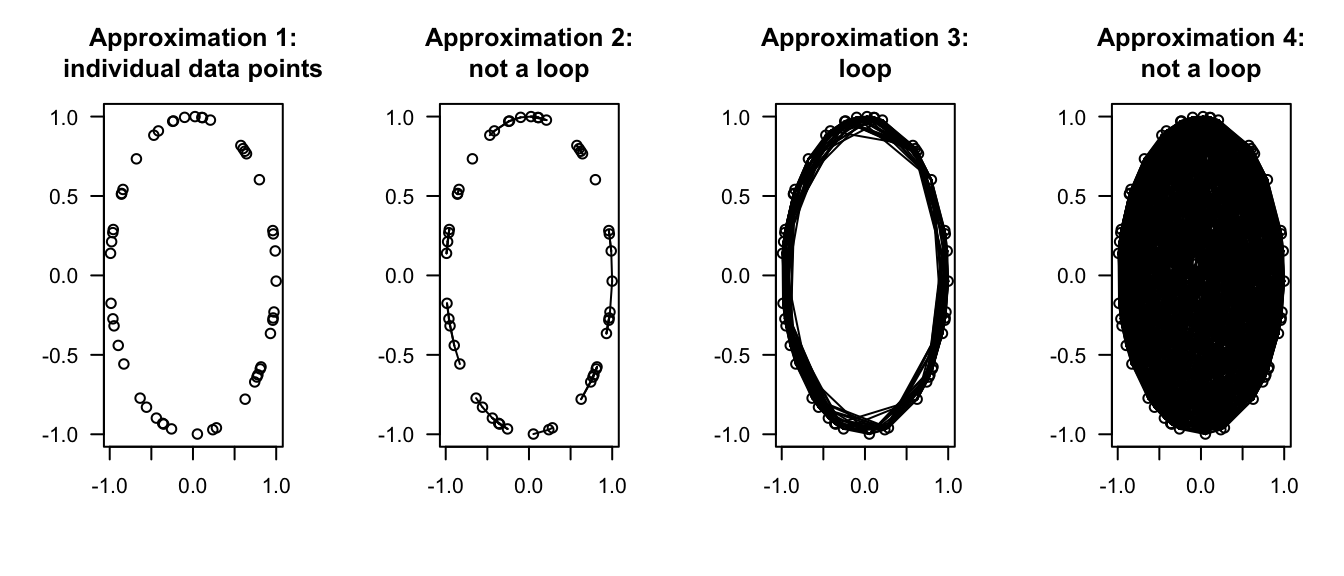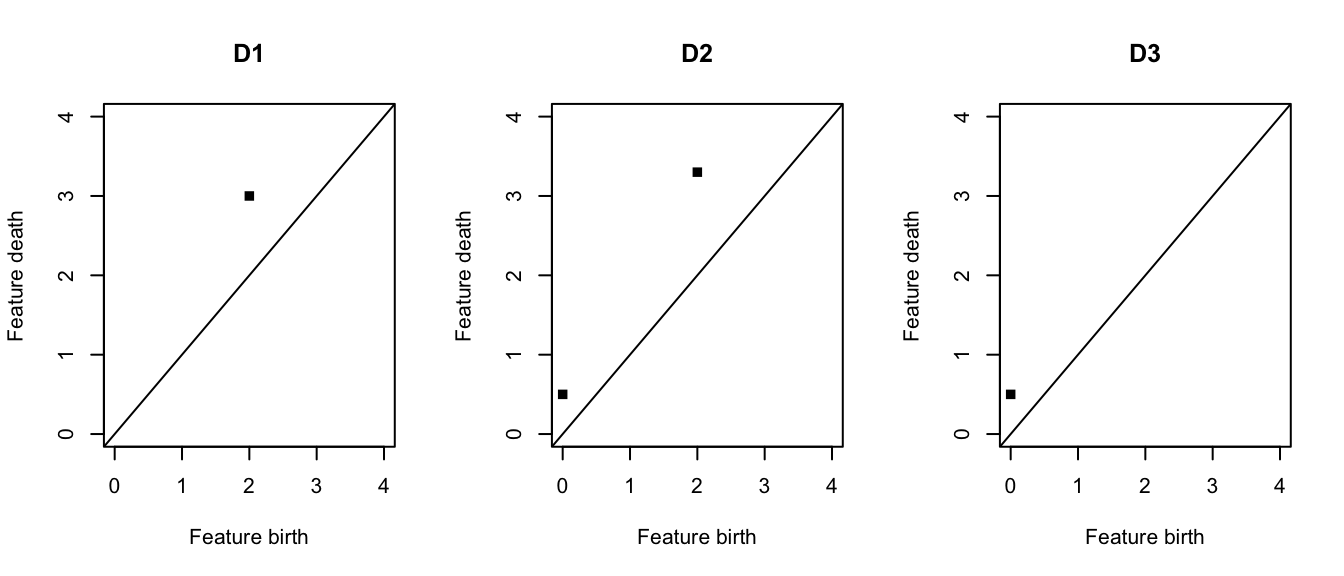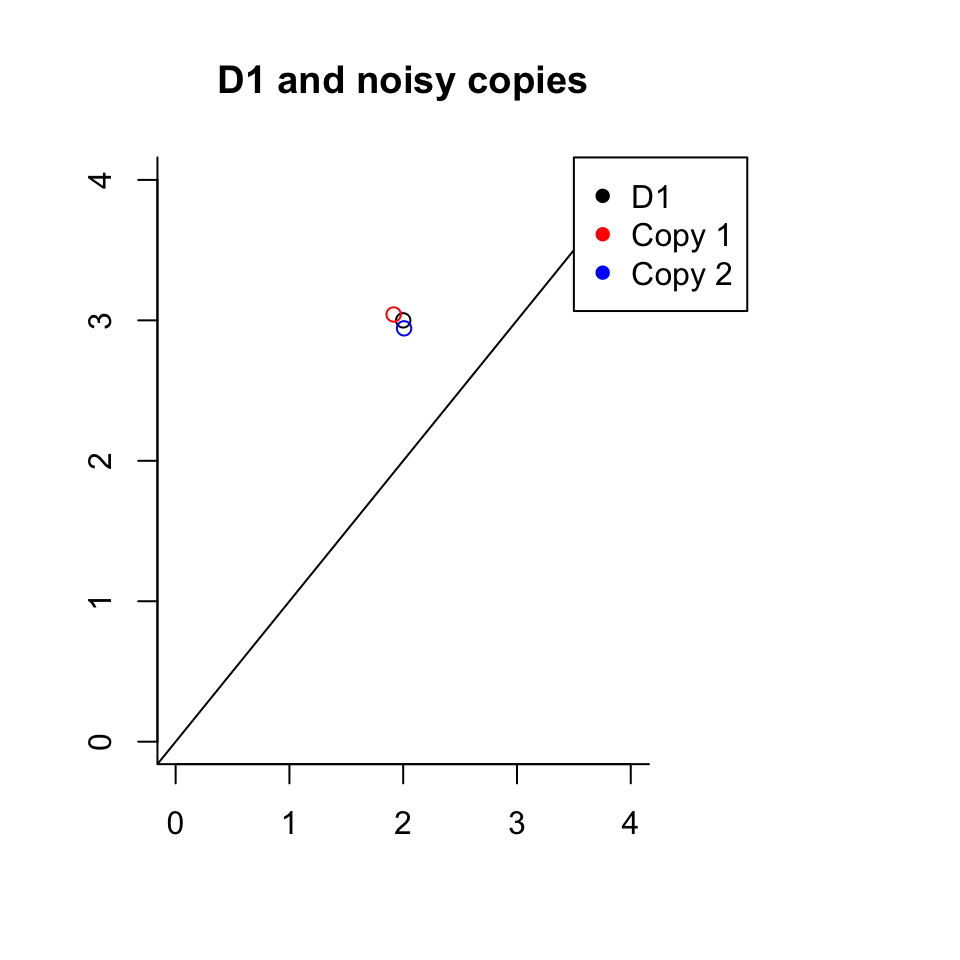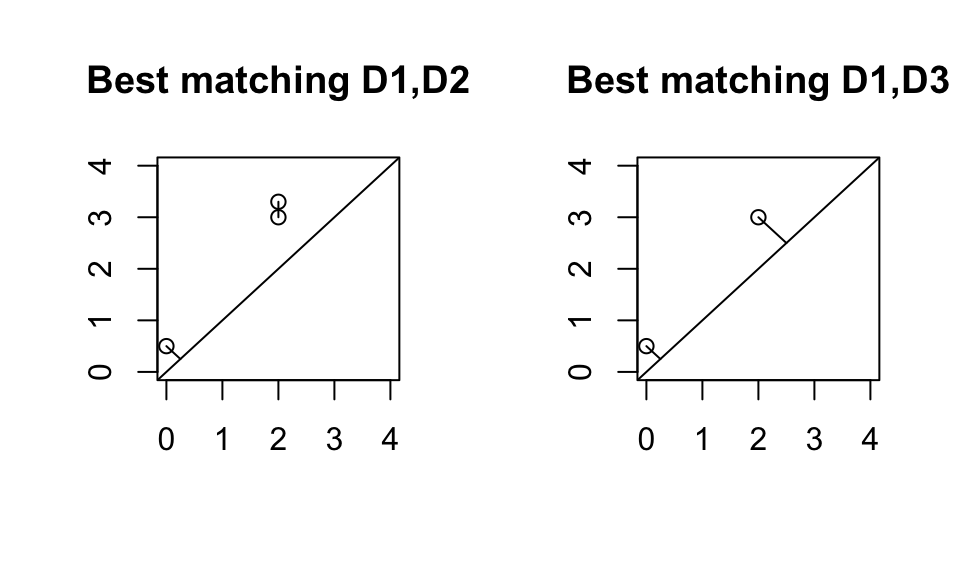# Introduction

Topological data analysis is a relatively new area of data science which can compare and contrast data sets via non-linear global structure. The main tool of topological data analysis, persistent homology (Edelsbrunner, Letscher, and Zomorodian 2000; Zomorodian and Carlsson 2005), builds on techniques from the field of algebraic topology to describe shape features present in a data set (stored in a “persistence diagram”). Persistent homology has been used in a number of applications, including

• distinguishing between the topology of human brain function of healthy control subjects vs. subjects with a neurological disorder (Gracia-Tabuenca et al. 2020; Hyekyoung et al. 2014; Lee et al. 2011),
• predicting stock market crashes from the topology of stock price correlations over time (Yen and Cheong 2021),
• finding non-trivial and complex topological structure in local patches of naturalistic images (Carlsson et al. 2007),
• translating sentences in one language into another language (from a set of candidate sentences) using the persistence diagrams of their word embeddings (Haim Meirom and Bobrowski 2022), and
• improving model predictions of various chemical properties of molecules by including topological features (Krishnapriyan 2021), etc.

For a broad introduction to the mathematical background and main tools of topological data analysis, see (Chazal and Michel 2017).

Distance and kernel functions are used to calculate differences and similarities respectively between objects in complicated spaces, and are the foundation of many machine learning algorithms which are used in traditional data science (Murphy 2012). Several papers have used distance and kernel computations tailored to persistence diagrams (Kerber, Morozov, and Nigmetov 2017; Le and Yamada 2018) in order to perform machine learning or inference tasks with groups of persistence diagrams, but to date no publicly available software in either R or python provides the functionality for these types of analyses.

Two main R packages exist for computing and comparing persistence diagrams – TDA (B. T. Fasy et al. 2021) and TDAstats (Wadhwa et al. 2019). While both packages have been used to carry out topological data analysis in R, neither package supports machine learning with groups of persistence diagrams because both lack a kernel function. TDAstats has an inference method; however, it is based on a non-standard distance function (making inferences drawn from this function unsubstantiated). TDA has a standard distance function but no inference function (and also stores persistence diagrams in a non-standard data structure, which requires additional preprocessing steps for further analysis).

In order to make the power of topological data analysis more readily available to data and machine learning practitioners, it would be very helpful to have a single software package that can

1. deliver a fast engine for calculating persistence diagrams,
2. convert persistence diagrams computed using the R packages TDA and TDAstats into a commonly-used data type for data analyses (a data frame),
3. implements fast versions of both distance and kernel calculations for pairs of persistence diagrams,
4. contribute tools for interpreting persistence diagrams, and
5. provide parallelized methods for machine learning and inference for persistence diagrams.

In accomplishing these goals TDApplied is a comprehensive and optimized package which permits researchers and data scientists to analyze multiple data sets using machine learning and inference via topological data analysis. This vignette documents the theory and application of TDApplied functions, on both simulated and neuroscience data.

# Software Review

Topological data analysis has gained popularity over the past two decades since the original paper on persistent homology was published (Edelsbrunner, Letscher, and Zomorodian 2000), and two main R packages have been created for topological data analysis:

1. The R package TDA (B. T. Fasy et al. 2021) is a wrapper for a number of C++ persistent homology packages, including Dionysus (Morozov 2017), GUDHI (Lacombe et al. 2019) and PHAT (Bauer, Kerber, and Reininghaus 2013), and provides both distance calculation capabilities between persistence diagrams and the ability to find representative data points of each topological feature in a persistence diagram (the points for each feature are called a “representative cycle”). Moreover, a method is provided for determining which topological features in a persistence diagram should be considered “real” from a statistical perspective.
2. The R package TDAstats (Wadhwa et al. 2019) is a wrapper of the C++ persistent (co)homology engine Ripser (Bauer 2015; Silva and Vejdemo-Johansson 2011b), and provides an inference method for detecting group differences of two groups of persistence diagrams (as in (Robinson and Turner 2017). However, the distance calculation currently implemented in that package, is not based on an accepted distance metric for persistence diagrams.

The paper (Somasundaram et al. 2021) compared the runtimes of the homology calculations in the R packages TDA and TDAstats, and concluded that certain persistent homology calculations are faster with TDAstats. The choice of which package to use therefore may vary by application, depending on whether speed is desired or whether knowledge of which data points represent which topological feature. Therefore, TDApplied can accept as input persistence diagrams computed from either package in order to cover a wide variety of potential use-cases.

It is worthwhile to note that there are two other R packages for carrying out topological data analysis - TDAvec (Islambekov and Luchinsky 2022) (methods for vectorizing persistence diagrams) and TDAkit (You and Yu 2021) (clustering and dimension reduction methods for functional summaries of persistence diagrams, called persistence landscapes/silhouettes). Topological summaries like persistence landscapes have their own suite of statistical methods (Bubenik 2015; Bubenik and Dlotko 2017) due to their simpler underlying space compared to that of persistence diagrams (Robinson and Turner 2017). There are also several python packages dedicated to topological data analysis calculations, including scikit-TDA (Saul and Tralie 2019) (which computes persistence diagrams, and distances/kernels between them, using several python libraries), and giotto-tda (Tauzin et al. 2020) (which computes persistence diagrams and uses them to analyze time series data).

There are a number of shortcomings of available topological data analysis software. Firstly, in both python and R there is currently no package which allows for machine learning and inference of persistence diagrams, a limitation which greatly constrains the types of analyses that can be carried out. In R this is partially because there is no package for kernel calculations of persistence diagrams, but the very slow computation of distances between persistence diagrams in the TDA package also inhibits the practicality of distance-based inference procedures. Additionally, in R, the output of persistent homology calculations from the package TDA is a list with an element called “diagram” of class “diagram,” which is not compatible with data frame methods that form the basis for data analysis in R. On the other hand, TDAstats does not compute a published distance metric for persistence diagrams, making inferences drawn from its permutation_test function unclear. Overall, the non-standard data type returned by persistent homology calculations in TDA, the slow distance calculations in TDA and the non-published distance metric in TDAstats may be limiting the development of TDA applications in R.

# Package TDApplied

The package TDApplied aims to solve the five goals outlined in the introductory paragraph. Firstly, the function diagram_to_df allows the conversion of the output of TDA persistent homology calculations to a data frame. Secondly, the functions diagram_distance and diagram_kernel allow for fast distance and kernel calculations respectively, and their counterparts distance_matrix and gram_matrix compute in parallel, for scalability, the (cross) distance and (cross) Gram matrices respectively. Thirdly, these distance and kernel calculations are used to perform machine learning and inference on persistence diagrams. Methods include

1. dimension reduction with metric multidimensional scaling (MDS) and kernel principal components analysis (kpca),
2. clustering with kernel k-means,
3. regression and classification with kernel support-vector machines (ksvm), and
4. inference with distance and kernel calculations looking for group differences and group independence, respectively.

The kernel machine learning methods implemented in TDApplied are wrappers of the flexible R package for kernel calculations kernlab (Karatzoglou et al. 2004), with some additional processing steps specific to persistence diagrams. Fourthly, a fast method for computing persistence diagrams via python is implemented in the PyH function. Finally, interpretative tools for persistence diagrams include the plot_diagram function for plotting persistence diagrams and the bootstrap_persistence_thresholds function for determining which topological features in a dataset should be considered “real.” In the subsequent sections we will describe these applications in more detail.

# Background: Persistent Homology

The main tool of topological data analysis is called persistent homology (see (Edelsbrunner, Letscher, and Zomorodian 2000) for the introductory paper, and (Zomorodian and Carlsson 2005) for further computational details). Persistent homology has been applied in a variety of areas, including (but not limited to) economics (largely for the application of time series, for example see (Yen and Cheong 2021)), neuroscience (see (al 2021) for a number of functional MRI applications), etc.

Persistent homology starts with data points and a distance function. It assumes that these points were sampled from some kind of shape. This shape has certain features that exist at various scales, but sampling induces noise in these features. Persistent homology aims to describe certain mathematical features of this underlying shape, by forming approximations to the shape at various distance scales. The mathematical features which are tracked are clusters (connected components), loops (ellipses), voids (spheres), etc, and the “significance” of each feature is calculated (i.e. are the feature “real” or not). The homological dimension of these features are 0, 1 and 2 respectively (higher dimensional features can also be calculated). What’s really interesting about these particular mathematical features is that they can tell us where our data is not, which is extremely important information which other data analysis methods can’t provide.The persistent homology algorithm proceeds in the following manner: first, if the input is a dataset and distance metric, then the distance matrix, storing the distance metric value of each pair of points in the dataset, is computed. Next, a parameter $$\epsilon \geq 0$$ is grown starting at 0, and at each $$\epsilon$$ value we compute a shape approximation of the dataset $$C_{\epsilon}$$, called a simplicial complex (see (Edelsbrunner, Letscher, and Zomorodian 2000) or (Zomorodian and Carlsson 2005) for more details). We construct $$C_{\epsilon}$$ by connecting all pairs of points whose distance is at most $$\epsilon$$. To encode higher-dimensional structure in these approximations, we also add a triangle between any triple of points which are all connected, a tetrahedron between any quadruple of points which are all connected, etc. Note that this process of forming a sequence of skeletal approximations is called a filtration, and other methods exist for forming the approximations (the one described here is the most commonly used, called the Rips-Vietoris complex).

At any given $$\epsilon$$ value, some topological features will exist in $$C_{\epsilon}$$. As $$\epsilon$$ grows, the $$C_{\epsilon}$$’s will contain each other, i.e. if $$\epsilon_{1} < \epsilon_{2}$$ then every edge (triangle, tetrahedron etc.) in $$C_{\epsilon_1}$$ will also be present in $$C_{\epsilon_2}$$. Therefore, each topological feature will be “born” at some $$\epsilon_{birth}$$ value, and “die” at some some $$\epsilon_{death}$$ value. Consider the example of a loop – a loop will be “born” when the last connection around the circumference of the loop is connected (at the $$\epsilon$$ value which is the largest distance between consecutive points around the loop), and the loop will “die” when the last connection across the loop’s diameter is connected thereby filling in its hole.

Therefore, the output of persistent homology, a persistence diagram, in each dimension has one 2D point for each topological feature found in the filtration process, where the $$x$$-value of the point is the birth $$\epsilon$$ value and the $$y$$-value is the death $$\epsilon$$ value. This is why every point lies above the diagonal – features die after they are born! The difference of a points $$y$$ and $$x$$ value, $$y-x$$, is called the “persistence” of the corresponding topological feature. Points which have high (large) persistence likely represent real topological features of the dataset, whereas points with low persistence likely represent topological noise.

A persistence diagram containing $$n$$ topological features can be represented in a vector of length $$2n$$. However, persistence diagrams can contain different numbers of features, and the ordering of the features is arbitrary. There, there is no obvious vector representation of all persistence diagrams that can be used as the input of machine learning or statistical inference. Nevertheless, we can apply a number of these techniques to persistence diagrams provided we can quantify how near (similar) or far (distant) they are from each other, and describing suitable distance and similarity measures with their accompanying analysis methods will be the content of the following section.

# TDApplied Methods and Underlying Theory

In this section we will describe the various computational tools implemented in the package TDApplied to analyze persistence diagrams, both explaining the mathematics and providing functional examples. To run our examples we must start by loading the TDApplied package:

library("TDApplied")

Since TDApplied uses the output of TDA/TDAstats calculations (or its own persistent homology function, “PyH,” discussed later) as inputs to its functions, either TDA or TDAstats should be at least installed (if not attached) when using TDApplied. All examples, except those in the subsection on bootstrapping, will analyze simple diagrams which are random deviations of three persistence diagrams called D1, D2 and D3 (each with points in dimension 0 only), which we will plot with the TDApplied function plot_diagram:

D1 = data.frame(dimension = c(0),birth = c(2),death = c(3))
D2 = data.frame(dimension = c(0),birth = c(2,0),death = c(3.3,0.5))
D3 = data.frame(dimension = c(0),birth = c(0),death = c(0.5))
par(mfrow = c(1,3))
plot_diagram(D1,title = "D1",max_radius = 4,legend = F)
plot_diagram(D2,title = "D2",max_radius = 4,legend = F)
plot_diagram(D3,title = "D3",max_radius = 4,legend = F)When desired, random Gaussian noise with a small variance will be added to the birth and death values of the points in these three diagrams (being careful make sure the points always have appropriate birth and death values), which will be achieved with a function (only used in this vignette), generate_TDApplied_vignette_data.

Here is an example of making noisy copies of D1:## Distance Between Persistence Diagrams

As the persistence diagram is a descriptor of the underlying shape structure of a dataset, it can be useful to quantify the differences between pairs of persistence diagrams. There are several ways to compute distances between persistence diagrams in the same homological dimension (like dimension 0 for clusters, dimension 1 for loops, etc.). The most common two are called the 2-wasserstein and bottleneck distances (Kerber, Morozov, and Nigmetov 2017). These techniques find an optimal matching of the 2D points in their input two diagrams, and compute a cost of that optimal matching. A point from one diagram is allowed either to be paired (matched) with a point in the other diagram or its diagonal projection, i.e. the nearest point on the diagonal line $$y=x$$ (matching a point to its diagonal projection is essentially saying that feature is likely topological noise because it died very soon after it was born).

Allowing points to be paired with their diagonal projections both allows for matchings of persistence diagrams with different numbers of points (which is almost always the case in practice) and also formalizes the idea that some points in a persistence diagram represent noise. The “cost” value associated with a matching is given by either (i) the maximum of infinity-norm distances between paired points, or (ii) the square-root of the sum of squared infinity-norm between matched points. The cost of the optimal matching under loss (i) is called the bottleneck distance of persistence diagrams, and the cost of the optimal matching of cost (ii) is called the 2-wasserstein metric of persistence diagrams. Both distance metrics have been used in a number of applications, but the 2-wasserstein metric is able to find more fine-scale differences in persistence diagrams compared to the bottleneck distance. The problem of finding an optimal matching can be solved with the Hungarian algorithm, which is implemented in the R package clue (Hornik 2005).In the picture we can see that there is a “better” matching between D1 and D2 compared to D1 and D3, so the (wasserstein/bottleneck) distance value between D1 and D2 would be smaller than that of D1 and D3.

The wasserstein and bottleneck distances have been implemented in the TDApplied function diagram_distance. We can confirm that the distance between D1 and D2 is smaller than D1 and D3 for both distances:

# calculate 2-wasserstein distance between D1 and D2
diagram_distance(D1,D2,dim = 0,p = 2,distance = "wasserstein")
#>  0.3905125

# calculate 2-wasserstein distance between D1 and D3
diagram_distance(D1,D3,dim = 0,p = 2,distance = "wasserstein")
#>  0.559017

# calculate bottleneck distance between D1 and D2
diagram_distance(D1,D2,dim = 0,p = Inf,distance = "wasserstein")
#>  0.3

# calculate bottleneck distance between D1 and D3
diagram_distance(D1,D3,dim = 0,p = Inf,distance = "wasserstein")
#>  0.5

There is a generalization of the 2-wasserstein distance for any $$p \geq 1$$, the $$p$$-wasserstein distance, which can also be computed using the diagram_distance function by varying the parameter p. To see the computational details and proof of correctness for wasserstein and bottleneck distance calculations, see the appendix.

Another distance metric between persistence diagrams, which will be useful for kernel calculations, is called the Fisher information metric, $$d_{FIM}(D_1,D_2,\sigma)$$ (details can be found in (Le and Yamada 2018)). The idea is to represent the two persistence diagrams as probability density functions, with a 2D-Gaussian point mass centered at each point in both diagrams (including the diagonal projections of the points in the opposite diagram), all of variance $$\sigma^2 > 0$$, and calculate how much those distributions agree on their pdf value at each point in the plane (called their Fisher information metric).